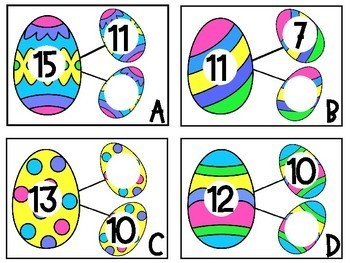digital

# Easter Themed Math StationsSubject
Resource Type
Formats

TpT Digital Activity

PDF (2 MB|27 pages)
Standards
\$2.00
TpT Digital Activity
Add notes & annotations through an interactive layer and assign to students via Google Classroom.
• Product Description
• Standards

A Basket Full of Easter Math Stations

This pack includes 4 games that can be used in math stations with a fun Easter theme.

Egg-cellent Missing Parts- A game that has number bonds with a missing part. The numbers are within 20. A recording sheet is included

Adding with my PEEPS- This activity has students adding 3 addends. The sums are within 20. A recording sheet is included.

Base Ten Bunnies- This activity helps students practice representing numbers within 100 using base ten and expanded form. A recording sheet is included.

Spring Missing Numbers- This activity allows students to use their number sense to fill in missing numbers within 120.

Enjoy!

to see state-specific standards (only available in the US).
Determine the unknown whole number in an addition or subtraction equation relating three whole numbers. For example, determine the unknown number that makes the equation true in each of the equations 8 + ? = 11, 5 = ▯ - 3, 6 + 6 = ▯.
Add and subtract within 20, demonstrating fluency for addition and subtraction within 10. Use strategies such as counting on; making ten (e.g., 8 + 6 = 8 + 2 + 4 = 10 + 4 = 14); decomposing a number leading to a ten (e.g., 13 - 4 = 13 - 3 - 1 = 10 - 1 = 9); using the relationship between addition and subtraction (e.g., knowing that 8 + 4 = 12, one knows 12 - 8 = 4); and creating equivalent but easier or known sums (e.g., adding 6 + 7 by creating the known equivalent 6 + 6 + 1 = 12 + 1 = 13).
Solve word problems that call for addition of three whole numbers whose sum is less than or equal to 20, e.g., by using objects, drawings, and equations with a symbol for the unknown number to represent the problem.
Understand that the two digits of a two-digit number represent amounts of tens and ones. Understand the following as special cases:
Total Pages
27 pages
N/A
Teaching Duration
N/A
Report this Resource to TpT
Reported resources will be reviewed by our team. Report this resource to let us know if this resource violates TpT’s content guidelines.
• Ratings & Reviews
• Q & A

Teachers Pay Teachers is an online marketplace where teachers buy and sell original educational materials.